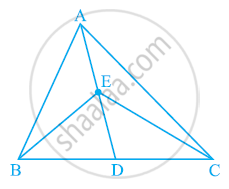Share

# In the given figure, E is any point on median AD of a ΔABC. Show that ar (ABE) = ar (ACE) - CBSE Class 9 - Mathematics

ConceptTriangles on the Same Base and Between the Same Parallels

#### Question

In the given figure, E is any point on median AD of a ΔABC. Show that ar (ABE) = ar (ACE)#### Solution

AD is the median of ΔABC. Therefore, it will divide ΔABC into two triangles of equal areas.

∴ Area (ΔABD) = Area (ΔACD) ... (1)

ED is the median of ΔEBC.

∴ Area (ΔEBD) = Area (ΔECD) ... (2)

On subtracting equation (2) from equation (1), we obtain

Area (ΔABD) − Area (EBD) = Area (ΔACD) − Area (ΔECD)

Area (ΔABE) = Area (ΔACE)

Is there an error in this question or solution?

#### APPEARS IN

NCERT Solution for Mathematics Class 9 (2018 to Current)
Chapter 9: Areas of Parallelograms and Triangles
Ex. 9.30 | Q: 1 | Page no. 162

#### Video TutorialsVIEW ALL 

Solution In the given figure, E is any point on median AD of a ΔABC. Show that ar (ABE) = ar (ACE) Concept: Triangles on the Same Base and Between the Same Parallels.
S